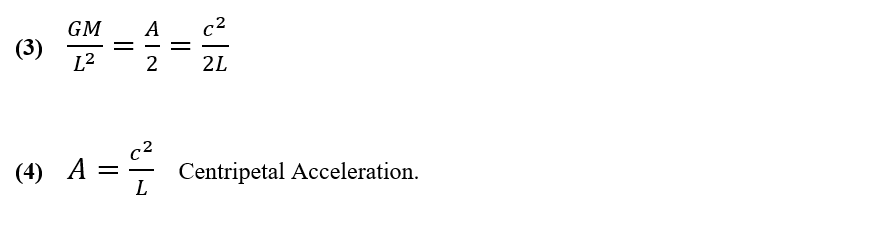Monday, 22 February 2021 17:06

## Equation 3 and 4Equations 3 and 4

Some commentators have described Centrifugal Forces as ‘fictitious’ or even non-existent forces. Stationary observers do not observe centrifugal forces, but in the reference frame of moving observers, centrifugal forces become very real.

If one observer spins a second (smaller!) observer around in a basket on the end of a rope, the stationary observer must exert a centripetal pull on the rope. The spun observer will feel a centrifugal push into the basket. The two equal and opposite forces balance but only one of them exists in each of the observer’s reference frames.

Equations 3 and 4 show the centripetal aspect of the balanced forces that maintain the orbits of structures around the inside of hyperspheres. Equation 4 provides a simple method of calculating antipode length L from acceleration A, or vice versa, and it also plays a part in the derivation of the Redshift - Distance Equation 6.

For the equal and opposite complimentary centrifugal force see equation 5.